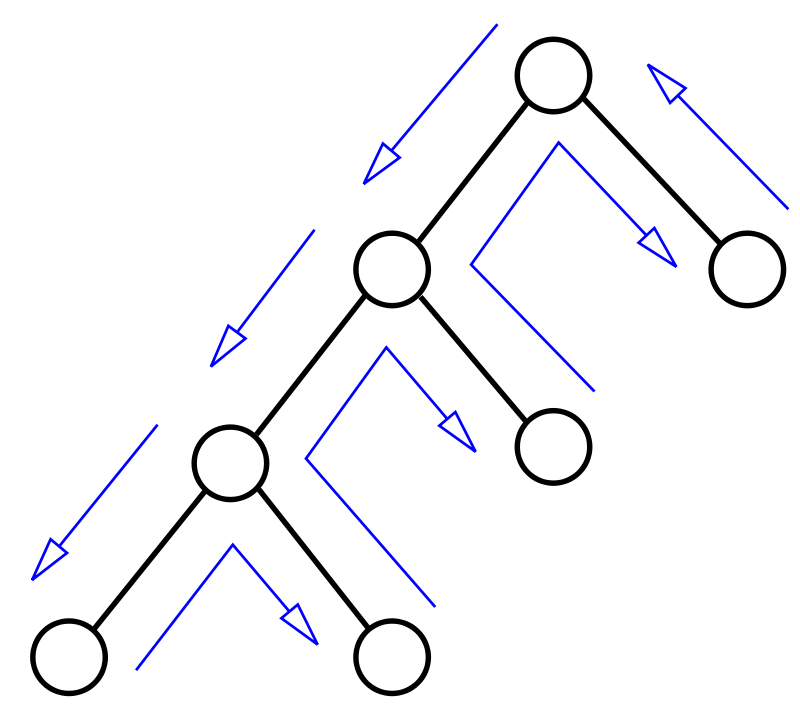2 views

I'm having trouble understanding the DPLL algorithm for checking the satisfiability of a sentence in propositional logic. http://books.google.co.in/books?id=4fyShrIFXg4C&pg=PA250&lpg=PA250&dq=DPLL+algorithm+from+artificial+intelligence+A+modern+approach&source=bl&ots=oOoZsT8KFd&sig=pdmyUsQZZWw76guWY9eFJKyNsH0&hl=en&sa=X&ei=vBFeUOf1EMLrrQeanoG4DQ&ved=0CD0Q6AEwAw#v=onepage&q&f=falseThis algorithm is taken from the book Artificial Intelligence A modern approach. I'm finding it really confusing with those many function recursions. In particular, what does the `EXTEND()` function do, and what's the purpose behind the recursive calls to `DPLL()` ?

by (108k points)

DPLL algorithm is basically a combination of three concepts:

• Backtracking

• Unit propagation

• Pure literal ruleAnswering your first question, the function call EXTEND(P, true, model) will return a new partial model in which P has true value.

And for your second question, the DPLL is essentially a backtracking algorithm, and that is the main idea behind the recursive calls.

The algorithm is building a solution while trying assignments, you have a partial solution that might prove successful or not-successful as you go on.

The basic idea of the algorithm is:

1. "Guess" a variable

2. Find all unit clauses created from the last assignment and assign the needed value

3. Iteratively retry step 2 until there is no change (found transitive closure)

4. If the current assignment cannot yield true for all clauses - fold back from recursion and retry a different assignment

5. If it can - "guess" another variable (recursively invoke and return to 1)

For a better understanding of the DPLL algorithm, refer the following link:

http://www.dis.uniroma1.it/~liberato/ar/dpll/dpll.html